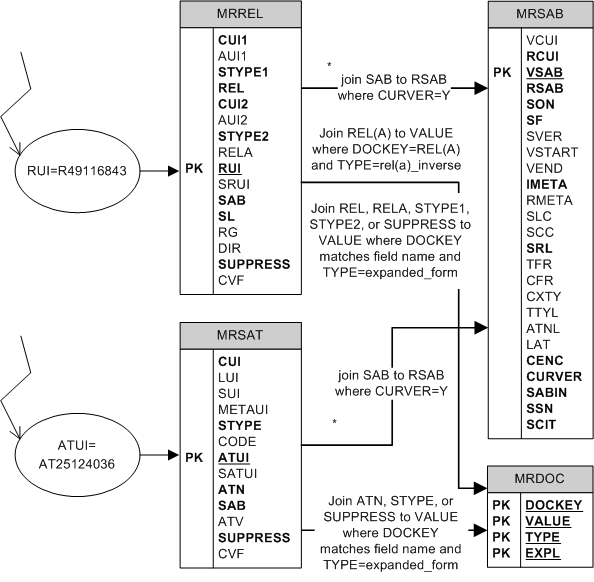U.S. Department of Health & Human Services

# UMLS Database Query Diagrams: How to find all information associated with the metadata of a particular relationship (RUI value) or attribute (ATUI value)This diagram shows how to find all information associated with the metadata of a particular relationship (RUI value) or attribute (ATUI value).

Searching for relationship STYPE1, STYPE2, or SUPPRESS values in MRDOC.RRF where DOCKEY is the field name will yield an expanded form of the abbreviated value of that field.

Two different types of information are found when searching for relationship REL values in MRDOC.RRF where the DOCKEY = 'REL.' One ("expanded_form") will yield an expanded form of the abbreviated REL value used within MRREL.RRF. The other ("rel_inverse") will yield the abbreviation of the inverse REL name (e.g., RB/RN). The inverse value itself will also always appear in MRDOC with "expanded_form" and "rel_inverse" entries (e.g., RN/RB).

Two different types of information are found when searching for relationship RELA values in MRDOC.RRF where the DOCKEY = 'RELA'. One ("expanded_form") will yield an expanded form of the abbreviated RELA value used within MRREL.RRF. The other ("rela_inverse") will yield the abbreviation of the inverse RELA name (e.g., has_part/part_of). The inverse value itself will also always appear in MRDOC.RRF with "expanded_form" and "rela_inverse" entries (e.g., part_of/has_part).

Searching for attribute ATN, STYPE, or SUPPRESS values in MRDOC.RRF where DOCKEY is the field name will yield an expanded form of the abbreviated value of that field.

Finally, searching for SAB values of either a relationship or attribute in MRSAB.RRF will yield detailed source provider information.

Corresponding Oracle Queries:

1. Find a relationship.

SELECT * FROM mrrel
WHERE rui = 'R49116843';

2. Find expanded form of a REL for a relationship.

SELECT a.expl FROM mrdoc a, mrrel b
WHERE b.rui = 'R49116843'
AND a.value = b.rel
AND a.type = 'expanded_form'
AND a.dockey = 'REL';

3. Find expanded form of a RELA for a relationship.

SELECT a.expl FROM mrdoc a, mrrel b
WHERE b.rui = 'R49116843'
AND a.value = b.rela
AND a.type = 'expanded_form'
AND a.dockey = 'RELA';

4. Find expanded form of a STYPE1 for a relationship.

SELECT a.expl FROM mrdoc a, mrrel b
WHERE b.rui = 'R49116843'
AND a.value = b.stype1
AND a.type = 'expanded_form'
AND a.dockey = 'STYPE1';

5. Find expanded form of a STYPE2 for a relationship.

SELECT a.expl FROM mrdoc a, mrrel b
WHERE b.rui = 'R49116843'
AND a.value = b.stype2
AND a.type = 'expanded_form'
AND a.dockey = 'STYPE2';

6. Find expanded form of a SUPPRESS for a relationship.

SELECT a.expl FROM mrdoc a, mrrel b
WHERE b.rui = 'R49116843'
AND a.value = b.suppress
AND a.type = 'expanded_form'
AND a.dockey = 'SUPPRESS';

7. Find source information for a relationship.

SELECT a.* FROM mrsab a, mrrel b
WHERE b.rui = 'R49116843'
AND a.rsab = b.sab
AND curver = 'Y';

8. Find inverse of a REL value

SELECT expl FROM mrdoc
WHERE type = 'rel_inverse'
AND dockey = 'REL'
AND value = 'RB';

9. Find inverse of a RELA value

SELECT expl FROM mrdoc
WHERE type = 'rela_inverse'
AND dockey = 'RELA'
AND value = 'part_of';

10. Find an attribute.

SELECT * FROM mrsat
WHERE atui = 'AT25124036';

11. Find expanded form of a ATN for an attribute.

SELECT a.expl FROM mrdoc a, mrsat b
WHERE b.atui = 'AT25124036'
AND a.value = b.atn
AND a.type = 'expanded_form'
AND a.dockey = 'ATN';

12. Find expanded form of a STYPE for an attribute.

SELECT a.expl FROM mrdoc a, mrsat b
WHERE b.atui = 'AT25124036'
AND a.value = b.stype
AND a.type = 'expanded_form'
AND a.dockey = 'STYPE';

13. Find expanded form of a SUPPRESS for an attribute.

SELECT a.expl FROM mrdoc a, mrsat b
WHERE b.atui = 'AT25124036'
AND a.value = b.suppress
AND a.type = 'expanded_form'
AND a.dockey = 'SUPPRESS';

14. Find source information for an attribute.

SELECT a.* FROM mrsab a, mrsat b
WHERE b.atui = 'AT25124036'
AND a.rsab = b.sab
AND curver = 'Y';